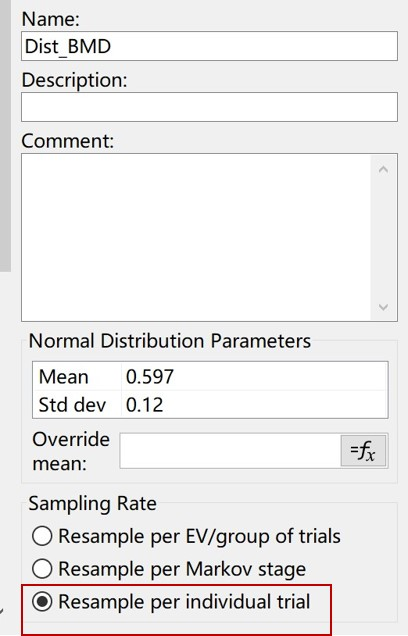# how to set up a probability distribution

Dear community,

I'm trying to do a cost-effectiveness analysis for a new osteoporosis screening tool. I plan to construct a logic for osteoporosis treatment if the BMD meets the reference value after an annual screening.

I plan to set up a probability distribution for baseline BMD and sample the baseline BMD of individual patients from the probability distribution in the microsimulation. can I ask how can I use TreeAge to implement this sample? Many thanks!

Zijie

Additionally, theoretically, each age group corresponds to a distribution. If my model cycles for 20 years, do I need to build 20 distributions? How to achieve a distribution corresponding to an age group?

• Thank you for the question. I can try and answer, but if you have a current license you may want to submit a query to support@treeage.com.

In terms of creating a distribution which you want to sample for each individual, you just need to select the "Resample per trial" radio button when you create the distribution. Any distribution you create can be sampled per individual.

In regards to the question about whether you need a different sample for each age group, that depends. Does probability change differently depending on age? It may not make sense to change the probability with every age. If you do want to change this, you would just need to create 20 distributions.

Let us know if you have more questions.

• Hi，Belinda

(1)I have already solved the first problem. I solved it through the following method, do you think it's correct?(2)Regarding the second question, I agree with your opinion. It may not make sense to change the probability with every age But it makes sense to change the probability within 10 years.

For example, let's assume the initial age is 60 years old. We are divided into three age groups, 60-70; 70-80；80+, corresponding to three different distributions.

60-70  Dist_BMD1

70-80  Dist_BMD2

80+   Dist_BMD3

Can we use the IF function to implement it as shown below?

if (Age<70, Dist_BMD1; (if Age>80; Dist_BMD3; Dist_BMD2))

Zijie

• That should work fine.

• Hi, Andrew

Many thanks.

Zijie